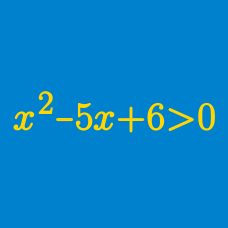Algebra

# Polynomial Inequalities

#### Challenge Quizzes

Find the range of $x$ that satisfies the inequality $x^2-14x+49 \geq 0.$

What is the quadratic inequality the solution of which is $x < -4$ or $x > 6?$

What is the solution to the following quadratic inequality where the constant $a$ is a negative number: $ax^2+12a^2x+32a^3 > 0?$

If the range of $x$ that satisfies the inequality $ax^2+35x-30 > 0$ can be expressed as $1 < x < b,$ what is $a+b?$

Which of the following integers does NOT satisfy the inequality $-3x^2+8x+35 > 0 ?$

×